# Modal Analysis of a Beam with Axial LoadThis test lets you check analysis results for a beam with a static preload. You will use a 1D mesh.Reference:

SFM, Afnor Technique, Guide de validation des prologiciels de calcul de structures, pp192-193.

##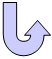Specifications

### Geometry Specifications

 Length: L = 2 m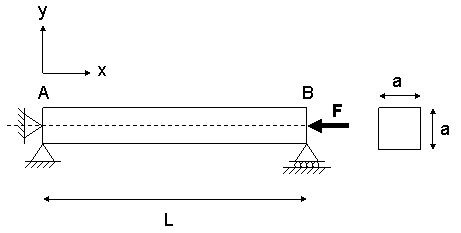Width and height: a = 0.05 m Area: 2.5e-3 m2 Inertia: Iz = 5.2083e-007 m4

### Analysis Specifications

 Young Modulus (material): E = 2e+011 N_m2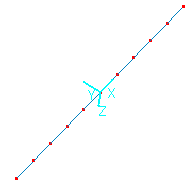Poisson's Ratio (material): ν = 0.3 Density: ρ = 7800 kg/m3 Mesh Specifications: 10 elements Restraints (User-defined): A: Tx = Ty = 0 B: Ty = 0 Loads: The distributed force Fx is a static pressing preload in the axis of the beam. There is two cases of preloading: |Fx| = 0 |Fx| = 105 N

##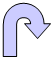Results

• The analytical solution is: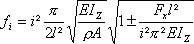• The table below presents the analysis results. Relative errors are listed:

 Load Fundamental natural mode of vibration Frequencies [Hz] Relative errors [%] Analytical results Computed results |Fx|=0 Bending mode 1 28.702 28.694 0.03 Bending mode 2 114.807 114.701 0.09 |Fx|=105N Bending mode 1 22.434 22.428 0.03 Bending mode 2 109.08 108.981 0.09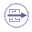To Perform the Test:

The Modal_analysis_beam_with_axial_load.CATAnalysis document presents a complete analysis of this case, computed with a load of 105 N.

To compute this case, proceed as follow:

1. Open the CATAnalysis document.

2. Compute the case and generate an image called Deformed mesh.

To compute the unloaded case, change in this CATAnalysis the value of the distributed force.